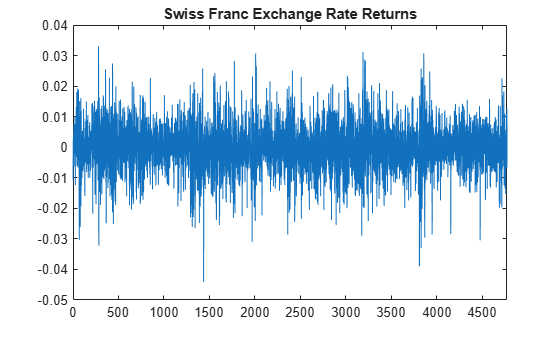Compare Conditional Variance Models Using Information Criteria

This example shows how to specify and fit a GARCH, EGARCH, and GJR model to foreign exchange rate returns. Compare the fits using AIC and BIC.

Load the foreign exchange rate data included with the toolbox. Convert the Swiss franc exchange rate to returns.

y = DataTable.CHF;
r = price2ret(y);
T = length(r);

logL = zeros(1,3); % Preallocate
numParams = logL;  % Preallocate

figure
plot(r)
xlim([0,T])
title('Swiss Franc Exchange Rate Returns')The returns series appears to exhibit some volatility clustering.

Step 2. Fit a GARCH(1,1) model.

Specify, and then fit a GARCH(1,1) model to the returns series. Return the value of the loglikelihood objective function.

Mdl1 = garch(1,1);
[EstMdl1,EstParamCov1,logL(1)] = estimate(Mdl1,r);

GARCH(1,1) Conditional Variance Model (Gaussian Distribution):

Value       StandardError    TStatistic      PValue
__________    _____________    __________    __________

Constant    1.6325e-06     4.3803e-07         3.727      0.00019376
GARCH{1}       0.91362      0.0068955         132.5               0
ARCH{1}       0.058566      0.0050049        11.702      1.2497e-31
numParams(1) = sum(any(EstParamCov1)); % Number of fitted parameters

Step 3. Fit an EGARCH(1,1) model.

Specify, and then fit an EGARCH(1,1) model to the returns series. Return the value of the loglikelihood objective function.

Mdl2 = egarch(1,1);
[EstMdl2,EstParamCov2,logL(2)] = estimate(Mdl2,r);

EGARCH(1,1) Conditional Variance Model (Gaussian Distribution):

Value      StandardError    TStatistic      PValue
_________    _____________    __________    __________

Constant        -0.29251       0.045942       -6.3669      1.9294e-10
GARCH{1}         0.96976      0.0046786        207.27               0
ARCH{1}          0.12292       0.012052        10.199      2.0113e-24
Leverage{1}    -0.013229      0.0049498       -2.6726       0.0075267
numParams(2) = sum(any(EstParamCov2));

Step 4. Fit a GJR(1,1) model.

Specify, and then fit a GJR(1,1) model to the returns series. Return the value of the loglikelihood objective function.

Mdl3 = gjr(1,1);
[EstMdl3,EstParamCov3,logL(3)] = estimate(Mdl3,r);

GJR(1,1) Conditional Variance Model (Gaussian Distribution):

Value       StandardError    TStatistic      PValue
__________    _____________    __________    __________

Constant       1.6543e-06     4.4107e-07        3.7506      0.00017638
GARCH{1}          0.91356       0.007004        130.43               0
ARCH{1}           0.05725      0.0067079        8.5347      1.4053e-17
Leverage{1}     0.0020101      0.0071432       0.28139         0.77841
numParams(3) = sum(any(EstParamCov3));

The leverage term in the GJR model is not statistically significant.

Step 5. Compare the model fits using AIC and BIC.

Calculate the AIC and BIC values for the GARCH, EGARCH, and GJR model fits. The GARCH model has three parameters; the EGARCH and GJR models each have four parameters.

[aic,bic] = aicbic(logL,numParams,T)
aic = 1×3
104 ×

-3.3329   -3.3321   -3.3327

bic = 1×3
104 ×

-3.3309   -3.3295   -3.3301

The GARCH(1,1) and EGARCH(1,1) models are not nested, so you cannot compare them by conducting a likelihood ratio test. The GARCH(1,1) is nested in the GJR(1,1) model, however, so you could use a likelihood ratio test to compare these models.

Using AIC and BIC, the GARCH(1,1) model has slightly smaller (more negative) AIC and BIC values. Thus, the GARCH(1,1) model is the preferred model according to these criteria.